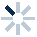# 3.6.4　python编程解决数学问题1、爱因斯坦的数学题

for i in range(0,500):
if i%2==1 and i%3==2 and i%5==4 and i%6==5 and i%7==0:
print("满足条件台阶为：",i)
1. 运行上述程序，满足上述条件的数是（　　　）*【多选题】
A.118
B.119
C.328
D.329
２、一汽车某时刻的里程数为 95859 ，一小时后里程表上出现了一个新的对称数（仍为五位数），问新的对称数是什么？2. 运行上述程序，满足条件的数是（　　）*
3、舍罕王的失算：传说舍罕王打算重赏 象棋的发明人宰相西萨班达依尔。国王问他有何要求，这位聪明的大臣 “胃口”看来并不大，他跪着说：“陛下，请您在这个棋盘的第一个小 格内，赏我一粒麦子，在第二个小格内给两粒，第三个格内给四粒，按 照这样的比例关系，摆满棋盘上所有64格的麦粒。

sum = 0
for i in range (1,65):
sum += 2 ** (i-1)　　　　#等同于sum=sum+2**(i-1)
print(sum)

３、30个人吃饭总共花了50元，每个男人吃饭要花 3元，每个女人吃饭要花 2元，小孩花 1元，问男人女人小孩各有多少人

for x in range(0,17):
for y in range(0,26):
if 3*x+2*y+30-x-y == 50:
print(f"男人：{x}，女人：{y}，小孩：{30-x-y}")

４、猴子吃桃问题：

an=2*(a(n+1)+1)

total = 1
for day in range(9, 0, -1):
total = (total + 1) * 2
print(f"第{day}天的桃子数为：{total}")

５、鸡兔同笼：
《孙子算经》中就记载了这个有趣的问题：“今有雉兔同笼，上有三十五头，下有九十四足，问雉兔各几何？” 意思是，有若干只鸡和兔子关在同一个笼子里，从上面数有35个头，从下面数有94只脚，问：笼子中有多少只鸡？多少只兔子？

foot=94
for c in range(0,head+1): 　　　#c 鸡的个数 ,r兔的个数
if c*2+r*4==foot:
print(f"鸡有：{c}只，兔有：{r}只")
６、牛吃草问题：

s:代表固定牧场的大小（固定）
v:代表每天草生长的速度（固定）
t:代表可以吃多少天（变化）
n:代表需要多少只牛可以在t时间内吃完草（变化）s+v*t1=n1*t1  ----------(1)
s+v*t2=n2*t2  ----------(2)
(1)-(2)得：　　v（t1-t2）=n1t1-n2t2
v=(n1t1-n2t2)/(t1-t2)
s=n1t1-vt1

n1,t1,n2,t2=20,15,14,24　　　#赋已知初值

v=abs(n1*t1-n2*t2)/abs(t1-t2)
s=n1*t1-v*t1
n=28
t=s/(n-v)
print(f'{n}头牛吃完需要{t}时间')
７、递归应用:1）、定义一个函数 sum_numbers;
2 )、 能够接收一个 nums 的整数参数；
3）、计算 1 + 2+３ = num 的结果（方便理解，只循环调用３次）；